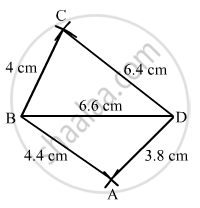Share

# Construct a Quadrilateral Abcd in Which Ab = 4.4 Cm, Bc = 4 Cm, Cd = 6.4 Cm, Da = 3.8 Cm and Bd = 6.6 Cm. - Mathematics

Course
ConceptConstructing a Quadrilateral When Two Diagonals and Three Sides Are Given

#### Question

Construct a quadrilateral ABCD in which AB = 4.4 cm, BC = 4 cm, CD = 6.4 cm, DA = 3.8 cm and BD= 6.6 cm.

#### Solution

$\text { First, we draw a rough sketch of the quadrilateral ABCD and write down its dimensions along the sides }.$

$\text { We may divide the quadrilateral into two constructible triangles ABD and BCD } .$

$\text { Steps of Construction: }$

$\text { Step I: Draw BD = 6 . 6 cm }$

$\text { Step II: With B as the centre and radius BC = 4 cm, draw an arc }.$

$\text { Step III: With D as the centre and radius 6 . 4 cm, draw an arc to intersect the arc drawn in Step II at C }.$

$\text { Step IV: With B as the centre and radius 4 . 4 cm, draw an arc on the side BD opposite to that of C }.$

$\text { Step V: With D as the centre and radius 3 . 8 cm, draw an arc to intersect the arc drawn in Step IV at A }.$

$\text { Step VI: Join BA, DA, BC and CD }$

$\text { The quadrilateral ABCD so obtained is the required quadrilateral } .$Is there an error in this question or solution?

#### APPEARS IN

RD Sharma Solution for Mathematics for Class 8 by R D Sharma (2019-2020 Session) (2017 to Current)
Chapter 18: Practical Geometry (Constructions)
Ex. 18.1 | Q: 1 | Page no. 4
Solution Construct a Quadrilateral Abcd in Which Ab = 4.4 Cm, Bc = 4 Cm, Cd = 6.4 Cm, Da = 3.8 Cm and Bd = 6.6 Cm. Concept: Constructing a Quadrilateral - When Two Diagonals and Three Sides Are Given.
S